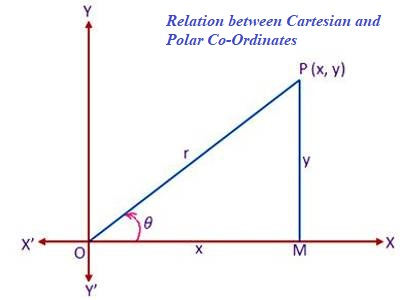Relation between Cartesian and Polar Co-Ordinates

Polar Co-ordinates are widely used in higher mathematics as well as in other branches of science. In mathematics, the polar coordinate system is a two-dimensional coordinate system in which each point on a plane is determined by a distance from a reference point and an angle from a reference direction.

Cartesian coordinates provide a method of rendering graphs and indicating the positions of points on a two-dimensional (2D) surface or in three-dimensional (3D) space. The two axes of two-dimensional Cartesian coordinates, conventionally denoted the x- and y-axes are chosen to be linear and mutually perpendicular.

Here we will learn to find the relation between Cartesian and Polar Co-Ordinates.

Let XOX’ and YOY’ be a set of rectangular Cartesian axes of polar Co-ordinates through the origin O. now, consider a polar Co-ordinates system whose pole and initial line coincide respectively with the origin O and the positive x-axis of the Cartesian system. Let P be any point on the plane whose Cartesian and polar Co-ordinates are (x, y) and (r, θ) respectively. Draw PM perpendicular to OX. Then we have,OM = x, PM = y, OP = r and < MOP = θ

Now, from the right-angled triangle MOP we get,

x/r = cos θ     or, x = r cos θ     …… (1)

and y/r = sin θ     or, y = r sin     …… (2)

Using (1) and (2) we can find Cartesian Co-ordinates (x, y) of the point whose polar Co-ordinates (r, θ) are given.

Again, from the right angled triangle OPM we get,

r² = x² + y²

or, r = √(x² + y²) …… (3)

and tan θ = y/x or, θ = tan[Math Processing Error]−1 y/x ……… (4)

Using (3) and (4) we can find the polar Co-ordinates (r, θ) of the points whose Cartesian Co-ordinates (x, y) are given.

Note:

If the Cartesian Co-ordinates (x, y) of a point are given then to find the value of the vectorial angle θ by the transformation equation θ = tan[Math Processing Error]−1 y/x we should note the quadrant in which the point (x, y) lies.

Examples of the relation between Cartesian and Polar Co-Ordinates.

The cartesian co-ordinates of a point are (- 1, -√3); find its polar co-ordinates.

Solution:

If the pole and initial line of the polar system coincide with the origin and positive x-axis respectively of the cartesian system and the cartesian and polar co-ordinates of a point are ( x, y ) and ( r, θ ) respectively, then

x = r cos θ and y= r sin θ.

In the given problem, x = -1 and y = -√3

Therefore, r cos θ = -1 and r sin θ = -√3

Therefore, r² Cos² θ + r² sin² = (- 1)² + (-√3)²

And tan θ = (r sin θ)/(r cos θ) = (-√3)/(-1) = √3 = tan π/3

Or, tan θ =tan(π+ π/3) [Since, the point (- 1, – √3) lise in the third quadrant]

Or, tan θ = tan 4π/3

Therefore, θ = 4π/3

Therefore, the polar co-ordinates of the point (- 1, – √3) are (2, 4π/3).

Information Source: